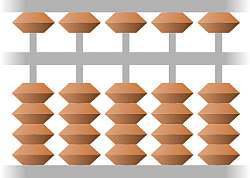# Cofactor Matrix Calculator

Instructions: Use this calculator to get compute the cofactor matrix associated to a given matrix that you provide. First, click on one of the buttons below to specify the dimension of the matrix.

Then, click on the first cell and type the value, and move around the matrix by pressing "TAB" or by clicking on the corresponding cells, to define ALL the matrix values.

\begin{bmatrix} & \\ & \end{bmatrix}

Cofactors are tightly associated with the inverse of a matrix, and they are stepping stone of the adjoint method used to compute the inverse of a matrix (when it exists).

Probably without knowing about, you have dealt with cofactors when computing a determinant of a matrix of 3x3 or larger. So, as you suspect, cofactors have to do with the determinants obtained when removing one row and one column.### How do you find the cofactor of a matrix?

The first thing is to compute the minors matrix. So, for a given n x n matrix $$A$$, the element in the i-th row and j-th column of the minors matrix is equal to the determinant of the sub-matrix formed by removing the i-th row and j-th column of the given matrix $$A$$.

So, if we call $$A[i,j]$$ to the sub-matrix obtained by removing the i-th row and the j-th column of $$A$$, formally we define the matrix of minors, $$M$$ as:

$M_{ij} = \det A[i,j]$

Notice that if $$A$$ is an n x n matrix, then $$M$$ is n x n as well.

### So, Whats a cofactor matrix?

Almost there. So the minors is the matrix that contains all these determinant of the corresponding sub-matrices obtained by deleting one row and one column. The cofactor is almost that, except that you add a sign (positive or negative), depending on the i and j.

Indeed, the cofactor matrix, $$C$$ is defined as:

$C_{ij} = (-1)^{i+j} M_{ij} = (-1)^{i+j} \det A[i,j]$

That looks pretty much like what you use when you compute determinants, huh? So, in order to compute the cofactor matrix, you need to compute a bunch of determinants.

### How to use this Cofactor matrix calculator with steps

In order to use this cofactor calculator, all you need to do is to provide the matrix $$A$$. The calculator will guide you through the process of computing the minors and the signs to get to the cofactors.### Example of cofactor Matrix calculation

Question: Assume you have the following matrix

$\begin{bmatrix} \displaystyle 1&\displaystyle 2&\displaystyle 1\\[0.6em]\displaystyle 2&\displaystyle 3&\displaystyle 1\\[0.6em]\displaystyle 1&\displaystyle 1&\displaystyle 2 \end{bmatrix}$

Solution:We need to compute the cofactor matrix of the $$3 \times 3$$ matrix that has been provided.

First we compute the minors matrix. We have that, by definition, the minors matrix $$M$$ is defined by the formula

$M_{ij} = \det A^{i,j}$

where in this case $$A^{i,j}$$ is the matrix $$A$$ after deleting row $$i$$ and column $$j$$.

Therefore, and based on the matrix $$A$$ provided we get the following coefficients of the minors matrix:

For $$A^{ 1, 1}$$:

$M_{ 1 1} = \det A^{ 1 1} = \begin{vmatrix} \displaystyle 3&\displaystyle 1\\[0.6em]\displaystyle 1&\displaystyle 2 \end{vmatrix} = 3 \cdot \left( 2 \right) - 1 \cdot \left(1 \right) = 5$

For $$A^{ 1, 2}$$:

$M_{ 1 2} = \det A^{ 1 2} = \begin{vmatrix} \displaystyle 2&\displaystyle 1\\[0.6em]\displaystyle 1&\displaystyle 2 \end{vmatrix} = 2 \cdot \left( 2 \right) - 1 \cdot \left(1 \right) = 3$

For $$A^{ 1, 3}$$:

$M_{ 1 3} = \det A^{ 1 3} = \begin{vmatrix} \displaystyle 2&\displaystyle 3\\[0.6em]\displaystyle 1&\displaystyle 1 \end{vmatrix} = 2 \cdot \left( 1 \right) - 1 \cdot \left(3 \right) = -1$

For $$A^{ 2, 1}$$:

$M_{ 2 1} = \det A^{ 2 1} = \begin{vmatrix} \displaystyle 2&\displaystyle 1\\[0.6em]\displaystyle 1&\displaystyle 2 \end{vmatrix} = 2 \cdot \left( 2 \right) - 1 \cdot \left(1 \right) = 3$

For $$A^{ 2, 2}$$:

$M_{ 2 2} = \det A^{ 2 2} = \begin{vmatrix} \displaystyle 1&\displaystyle 1\\[0.6em]\displaystyle 1&\displaystyle 2 \end{vmatrix} = 1 \cdot \left( 2 \right) - 1 \cdot \left(1 \right) = 1$

For $$A^{ 2, 3}$$:

$M_{ 2 3} = \det A^{ 2 3} = \begin{vmatrix} \displaystyle 1&\displaystyle 2\\[0.6em]\displaystyle 1&\displaystyle 1 \end{vmatrix} = 1 \cdot \left( 1 \right) - 1 \cdot \left(2 \right) = -1$

For $$A^{ 3, 1}$$:

$M_{ 3 1} = \det A^{ 3 1} = \begin{vmatrix} \displaystyle 2&\displaystyle 1\\[0.6em]\displaystyle 3&\displaystyle 1 \end{vmatrix} = 2 \cdot \left( 1 \right) - 3 \cdot \left(1 \right) = -1$

For $$A^{ 3, 2}$$:

$M_{ 3 2} = \det A^{ 3 2} = \begin{vmatrix} \displaystyle 1&\displaystyle 1\\[0.6em]\displaystyle 2&\displaystyle 1 \end{vmatrix} = 1 \cdot \left( 1 \right) - 2 \cdot \left(1 \right) = -1$

For $$A^{ 3, 3}$$:

$M_{ 3 3} = \det A^{ 3 3} = \begin{vmatrix} \displaystyle 1&\displaystyle 2\\[0.6em]\displaystyle 2&\displaystyle 3 \end{vmatrix} = 1 \cdot \left( 3 \right) - 2 \cdot \left(2 \right) = -1$

Summarizing, the minors matrix is:

$M = \begin{bmatrix} \displaystyle 5&\displaystyle 3&\displaystyle -1\\[0.6em]\displaystyle 3&\displaystyle 1&\displaystyle -1\\[0.6em]\displaystyle -1&\displaystyle -1&\displaystyle -1 \end{bmatrix}$

Now, we can compute the elements of the cofactor matrix $$C$$ using the formula

$C_{ij} = (-1)^{i+j}M_{ij}$

The above formula can be used directly because the minors are known already. We get

$C_{ 1 1} = (-1)^{ 1+1} \cdot 5 = (-1)^{ 2} \cdot 5 = 5$ $C_{ 1 2} = (-1)^{ 1+2} \cdot 3 = (-1)^{ 3} \cdot 3 = -3$ $C_{ 1 3} = (-1)^{ 1+3} \left(-1\right)= (-1)^{ 4} \left(-1\right) = -1$ $C_{ 2 1} = (-1)^{ 2+1} \cdot 3 = (-1)^{ 3} \cdot 3 = -3$ $C_{ 2 2} = (-1)^{ 2+2} \cdot 1 = (-1)^{ 4} \cdot 1 = -1$ $C_{ 2 3} = (-1)^{ 2+3} \left(-1\right)= (-1)^{ 5} \left(-1\right) = 1$ $C_{ 3 1} = (-1)^{ 3+1} \left(-1\right)= (-1)^{ 4} \left(-1\right) = 1$ $C_{ 3 2} = (-1)^{ 3+2} \left(-1\right)= (-1)^{ 5} \left(-1\right) = 1$ $C_{ 3 3} = (-1)^{ 3+3} \left(-1\right)= (-1)^{ 6} \left(-1\right) = 1$

Summarizing, the cofactor matrix is:

$C = \begin{bmatrix} \displaystyle 5&\displaystyle -3&\displaystyle -1\\[0.6em]\displaystyle -3&\displaystyle -1&\displaystyle 1\\[0.6em]\displaystyle 1&\displaystyle 1&\displaystyle 1 \end{bmatrix}$

which concludes the calculation.